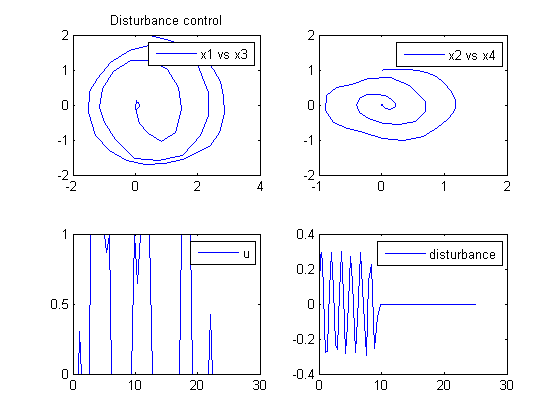31  Disturbance Control

Optimal On-Line Control and Classical Regulation Problem, Faina M. Kirillova, Institute of Mathematics National Academy of Sciences of Belarus.

Algorithm of Acting Optimal Controller

31.1  Problem Description

Find u over t in [0; 25 ] to minimize:

 J = 0

subject to:

 dx1 dt
= x3
 dx2 dt
= x4
 dx3 dt
= −x1+x2+u
 dx4 dt
= 0.1*x1−1.02*x2+0.3*sin(4*t)*(t<9.75)
 x(t0) = [0  0  2  1]
 x(tf) = [0  0  0  0]
 0 <= u <= 1

Reference: 

31.2  Problem setup

toms t
p = tomPhase('p', t, 0, 25, 80);
setPhase(p);

tomStates x1 x2 x3 x4
tomControls u

% Box constraints
cbox = {0 <= collocate(u) <= 1};

% Boundary constraints
cbnd = {initial({x1 == 0; x2 == 0
x3 == 2; x4 == 1})
final({x1 == 0; x2 == 0
x3 == 0; x4 == 0})};

% ODEs and path constraints
ceq = collocate({
dot(x1) == x3
dot(x2) == x4
dot(x3) == -x1+x2+u
dot(x4) == 0.1*x1-1.02*x2+0.3*sin(4*t).*(t<9.75)});

% Objective
objective = 0;

31.3  Solve the problem

options = struct;
options.name = 'Disturbance Control';
solution = ezsolve(objective, {cbox, cbnd, ceq}, [], options);
t  = subs(collocate(t),solution);
x1 = subs(collocate(x1),solution);
x2 = subs(collocate(x2),solution);
x3 = subs(collocate(x3),solution);
x4 = subs(collocate(x4),solution);
u  = subs(collocate(u),solution);
Problem type appears to be: lp
Starting numeric solver
===== * * * =================================================================== * * *
TOMLAB - Tomlab Optimization Inc. Development license  999001. Valid to 2011-02-05
=====================================================================================
Problem: ---  1: Disturbance Control            f_k       0.000000000000000000
sum(|constr|)      0.000000000046160833
f(x_k) + sum(|constr|)      0.000000000046160833
f(x_0)      0.000000000000000000

Solver: CPLEX.  EXIT=0.  INFORM=1.
CPLEX Dual Simplex LP solver
Optimal solution found

FuncEv  336 Iter  336
CPU time: 0.171875 sec. Elapsed time: 0.172000 sec.

31.4  Plot result

figure(1);
subplot(2,2,1)
plot(x1,x3,'-');
title('Disturbance control');
legend('x1 vs x3');
subplot(2,2,2)
plot(x2,x4,'-');
legend('x2 vs x4');

subplot(2,2,3)
plot(t,u,'-');
legend('u');

subplot(2,2,4)
plot(t,0.3*sin(4*t).*(t<9.75),'-');
legend('disturbance');